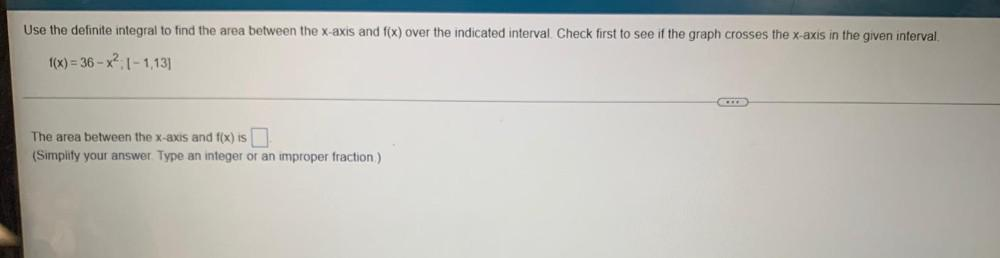Question:

# Use the definite integral to find the area between the x-axis and f(x) over the indicated interval. Check first to see if the grUse the definite integral to find the area between the x-axis and f(x) over the indicated interval. Check first to see if the graph crosses the x-axis in the given interval f(x) = 36 - X2: 1-1,13] The area between the x-axis and f(x) is (Simplify your answer. Type an integer or an improper fraction)